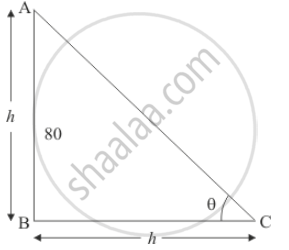Share

# Find the Angle of Elevation of the Sum (Sun'S Altitude) When the Length of the Shadow of a Vertical Pole is Equal to Its Height. - CBSE Class 10 - Mathematics

#### Question

Find the angle of elevation of the sum (sun's altitude) when the length of the shadow of a vertical pole is equal to its height.

#### Solution

Let θ be the angle of elevation of the sun. Let AB be the vertical pole of height h and BC be the shadow of equal length h.

Here we have to find the angle of elevation of the sun.

We have the corresponding figure as follows.So we use trigonometric ratios to find the required angle.

In a triangle ABC

=> tan theta = (AB/(BC)

=> tan theta = h/h

=> tan theta = 1

=> theta = 45^@`

Hence the angle of evevation of sun is  45°

Is there an error in this question or solution?

#### Video TutorialsVIEW ALL 

Solution Find the Angle of Elevation of the Sum (Sun'S Altitude) When the Length of the Shadow of a Vertical Pole is Equal to Its Height. Concept: Heights and Distances.
S